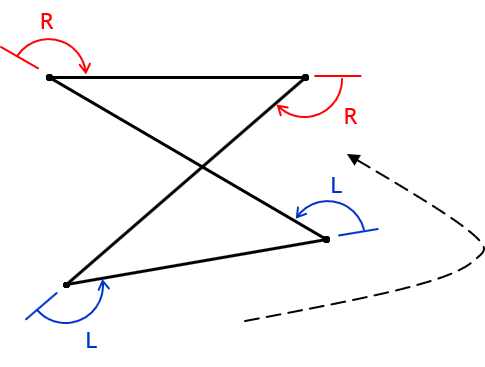## 2. Types of Horizontal Angles

### a. Interior/Exterior

On a closed non-crossing polygon, Figure G-5, horizontal angles can be either interior (red) or exterior (blue).Figure G-5 Interior and Exterior Angles

The geometric condition for a non-crossing polygon is Equation (G-1).Equation (G-1)

Interior and exterior don’t make sense if the polygon is open, Figure G-6(a), or if the polygon crosses itself, Figure G-6(b).a. Open Polygonb. Crossing Polygon Figure G-6 Interior/Exterior?

In the latter case, the polygon turns itself inside-out and Equation (E-1) does not apply. Open and crossing polygons need some other way to express an angle.

### b. Right/Left

Right or left is the rotational direction from the BS point to the FS point. Consider standing on the At point, looking at the BS point: an angle to the right means you physically turn your body to the right to see the FS point. An angle right is clockwise, left counter-clockwise, Figure G-7.Figure G-7 Clockwise/Counter-clockwise

Figure G-8(a) shows a closed non-crossing polygon with interior angles to the right; Figure G-8(b) to the left.a. Angles Rightb. Angles Left Figure G-8 Interior Angles

The dashed lines in Figure G-8 show the angle measurement sequence. In Figure G-8(a), interior angles to the right measurement progresses counter-clockwise around the polygon.

We can also have exterior angles to the right, Figure G-9(a), and to the left, Figure G-9(b).a. Angles Rightb. Angles Left Figure G-9 Exterior Angles

Right or left help with angle definitions on the open and crossing polygons. In Figure G-10, progressing in the direction of the dashed line, the open polygon has angles to the right.Figure G-10 Angles Right on Open Polygon

Figure G-11 shows a crossing polygon using angles left progressing in the direction of the dashed line.Figure G-11 Angles Right on Crossing Polygon

Note how the angles change from “interior” to “exterior” although there is no consistent polygon interior.

### c. Deflection Angles

A deflection angle is how much the next line deflects from an extension of the previous line. It consists of two parts: (1) magnitude and (2) direction. For example, in Figure G-12:

Line BC deflects from line AB 45° to the right, written as 45°R.

Line CD deflects from line BC 30°L.Figure G-12 Angles Right on Open Polygon

A deflection angle ranges from 0° (no deflection) to 180° (going back along the preceding line).

The mathematical angle condition for a closed non-crossing polygon, Figure G-13, is:Equation (G-2)

Right deflection angles are positive (+), left are negative (-).Figure G-13 Deflection Angles Around Polygon

Equation (G-2) is also the mathematical condition for a closed polygon which crosses itself an even number of times. For an odd number of crossings, Figure G-14, the mathematical condition is:Equation (G-3)Figure G-14 Odd Crossings Polygon

How does reversing travel direction around a polygon change the deflection angles? Figure G-15 shows that the deflection angle value is the same, but its direction is reversed.a. Clockwise Travel b. Counterclockwise Travel Figure G-15 Reversing Travel Direction